## Spherical Bessel Functions *

We will now give the full solutions in terms ofThese are written forbut can be are also valid forwhere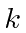becomes imaginary.The full regular solution of the radial equation for a constant potential for a givenis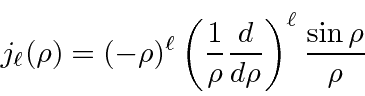the spherical Bessel function. For small, the Bessel function has the following behavior.The full irregular solution of the radial equation for a constant potential for a givenisthe spherical Neumann function. For small, the Neumann function has the following behavior.The lowestBessel functions (regular at the origin) solutions are listed below.The lowestNeumann functions (irregular at the origin) solutions are listed below.The most general solution is a linear combination of the Bessel and Neumann functions. The Neumann function should not be used in a region containing the origin. The Bessel and Neumann functions are analogous the sine and cosine functions of the 1D free particle solutions. The linear combinations analogous to the complex exponentials of the 1D free particle solutions are the spherical Hankel functions.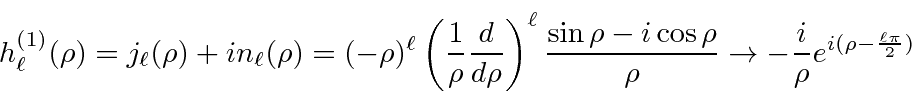The functional for for largeis given. The Hankel functions of the first type are the ones that will decay exponentially asgoes to infinity if, so it is right for bound state solutions.

The lowestHankel functions of the first type are shown below.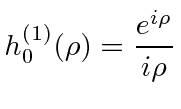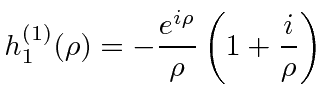We should also give the limits for large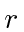,,of the Bessel and Neumann functions.Decomposing the sine in the Bessel function at large, we see that the Bessel function is composed of an incoming spherical wave and an outgoing spherical wave of the same magnitude.This is important. If the fluxes were not equal, probability would build up at the origin. All our solutions must have equal flux in and out.

Jim Branson 2013-04-22